# 「MoreThanJava」Day 2：变量、数据类型和运算符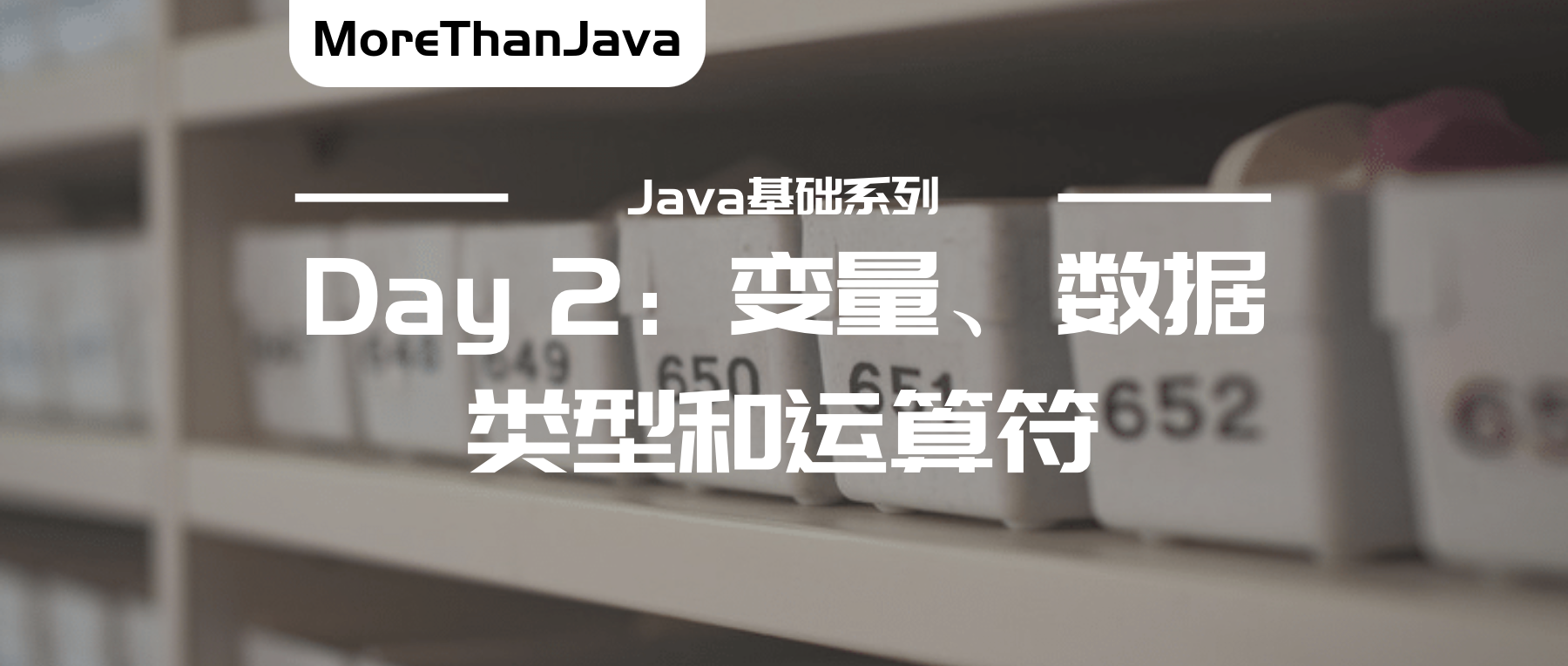• 「MoreThanJava」 宣扬的是 「学习，不止 CODE」，本系列 Java 基础教程是自己在结合各方面的知识之后，对 Java 基础的一个总回顾，旨在 「帮助新朋友快速高质量的学习」
• 当然 不论新老朋友 我相信您都可以 从中获益。如果觉得 「不错」 的朋友，欢迎 「关注 + 留言 + 分享」，文末有完整的获取链接，您的支持是我前进的最大的动力！

# Part 1. 数据类型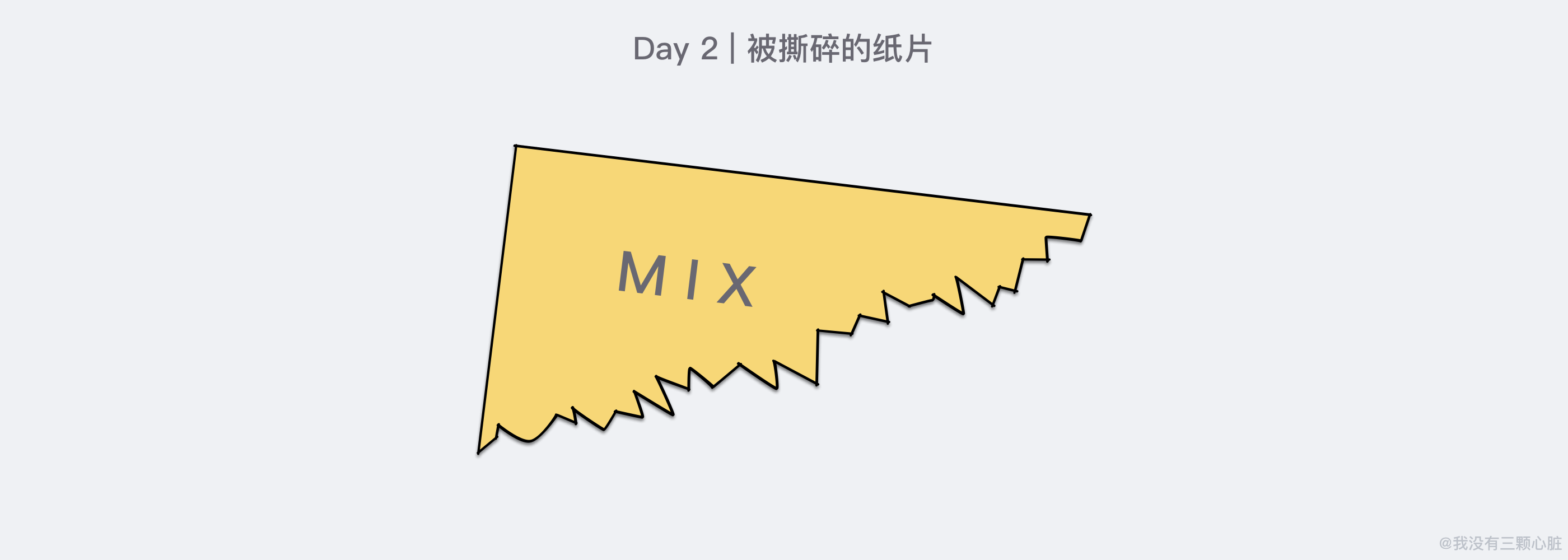## 8 种基本数据类型

Java 是一种强类型语言。这意味着必须为每一条数据声明一种类型。在 Java 中共有 8 种基本类型 (primitive type)*，其中 4 种整型、2 种浮点类型、1 种字符类型、1 种表示真值的 boolean *(布尔) 类型。

### 整型

int 4 字节 -2147483648 ~ 2147483647 (刚超过 20 亿，这个数值也就是 232 对半分成正负值，32=4字节*每个字节8位)
short 2 字节 -32768 ~ 32767
long 8 字节 -9223372036854775808 ~ 9223372036854775807
byte 1 字节 -128 ~ 127

### 浮点类型

float 4 字节 大约 ±3.40282347E + 38F (大约有效数为 6 ~ 7)
double 8 字节 大约 ±1.7976931486231580E + 308 (大约有效数为 15 位)

double 表示这种类型的数值精度是 float 类型的两倍 (也有人称 double 为双精度数值)

#### 浮点数精度问题System.out.println(0.1 + 0.2);

// 输出：0.30000000000000004

0.1 + 0.2 为什么会等于 0.30000000000000004？而不是我们想象中的 0.3

• 编程中的浮点数的精度往往都是有限的，单精度的浮点数使用 32 位表示，而双精度的浮点数使用 64 位表示；
• 数学中的小数系统可以通过引入无限序列….可以表示任意的实数；

0.3333333333333333....

0.10.2二进制 中同 1/3十进制 中一样，不属于整数的范畴，所以只能用近似值来代替，由于精度的限制 0.10.2 使用单精度浮点数表示的实际值为：0.1000000014901161193847656250.20000000298023223876953125，把它们相加起来得到的结果与我们在一开始看到的非常相似：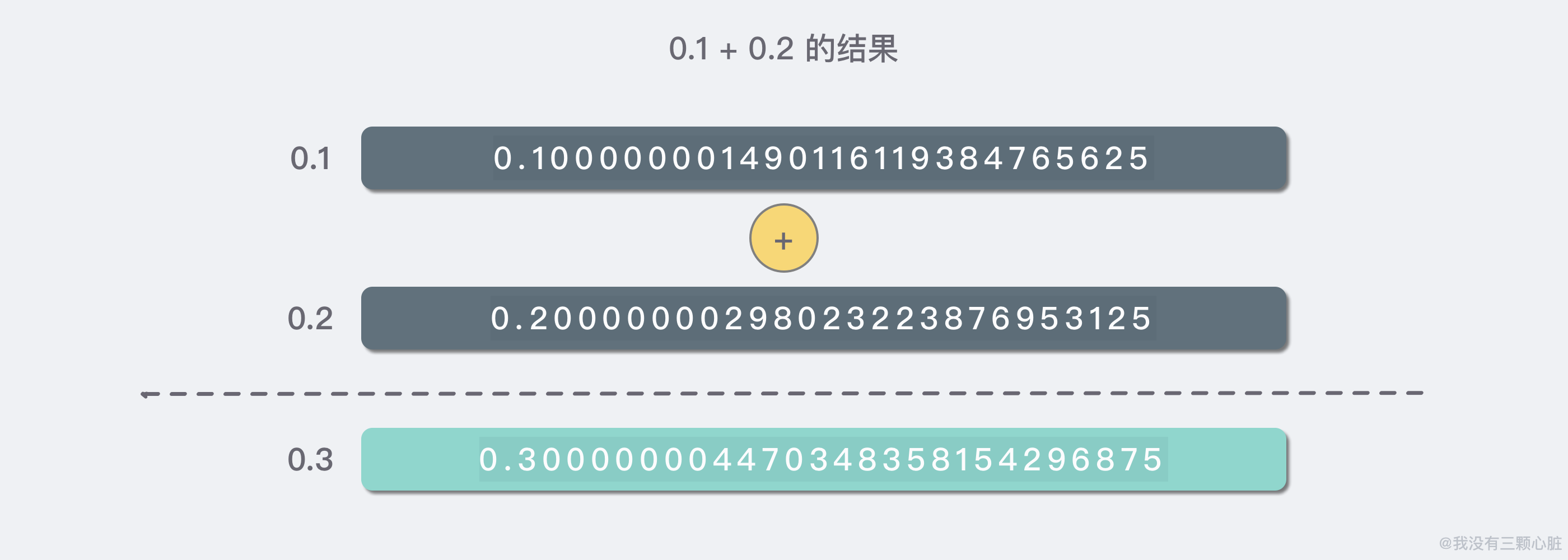• 使用二进制表达十进制的小数时，某些数字无法被有限位的二进制小数表示；
• 单精度和双精度的浮点数只包括 7 位或者 15 位的有效小数位，存储需要无限位表示的小数时只能存储近似值；

### char 类型

char 用来表示单个字符。在 Java 中 char 类型的数据使用 16 位来存储和表示，而许多编程语言则仅用 8 位。

char 类型的字面量值需要用 单引号 括起来。例如：'A' 是编码值为 65字符常量，它与 "A" 不同，"A" 是仅包含字符 A字符串 (String 类型)

(更多相关资料放入了下面的自取资料，感兴趣可以去阅读一下更多 char 类型的东西，不感兴趣跳过即可…)

### boolean 类型

boolean (布尔) 类型有两个值：falsetrue (注意这两个是布尔类型的字面常量也是保留字)，用来判断逻辑条件是否成立。

## 对象类型

Java 中的所有数据都属于「基本数据类型」或「对象」中的一种。

• 基本数据类型使用少量的固定字节数 (下面会详细介绍)
• 只有 8 种基本数据类型，您无法创建新的原始数据类型；
• 一个对象是一个较大的数据块，可能使用很多字节的内存；
• 对象类型的数据被称为
• Java 中已经封装了足够多的类用来满足您各类的需求，您也可以发明新的类来满足程序的特定需求；

# Part 2. 变量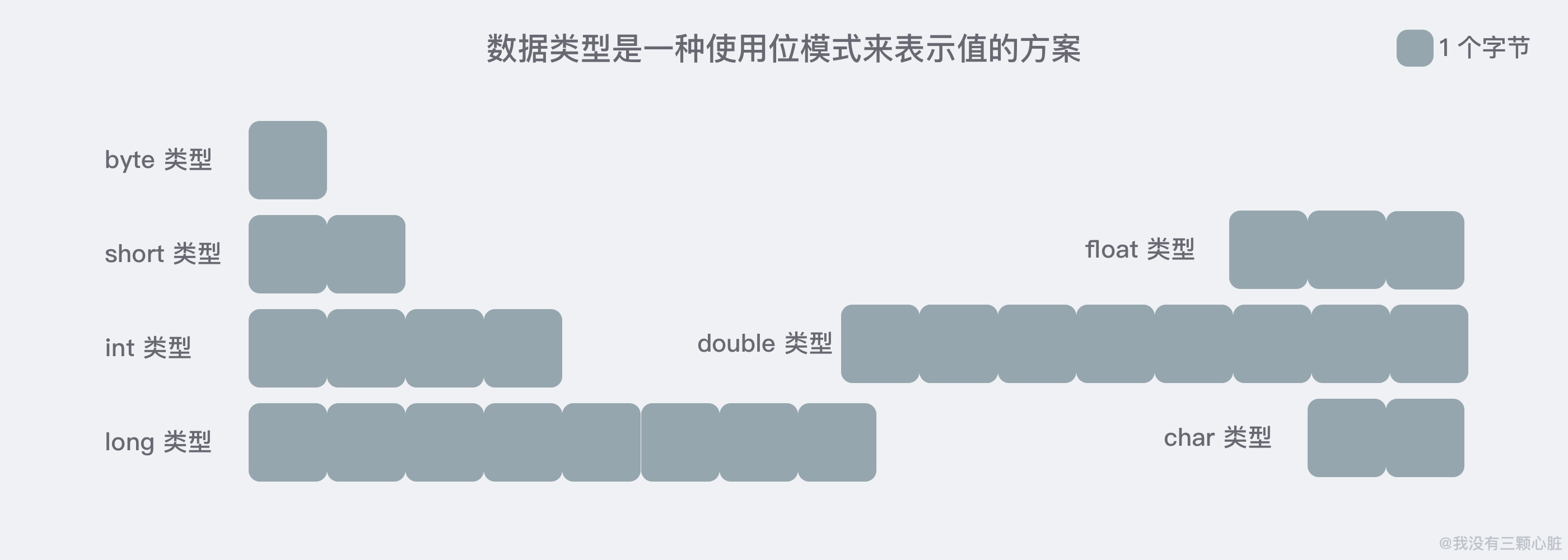## 声明变量

double salary;
int vacationDays;
long earthPopluation;
boolean finished;

### 变量命名

1. 变量名必须是一个以字母开头并由字母或数字构成的序列 (尽管 $ 是合法的，但不要在你自己的代码中使用这个字符，它只用在 Java 编译器或其他工具生成的名字中) 2. 每一个字符都有意义，且大小写敏感； 3. 不要使用 Java 中的保留字； #### 《阿里巴巴 Java 开发手册》规则 1. 【强制】 代码中的命名 (所有标识符) 均不能以下划线或美元符号开始，也不能以下划线或美元符号结束；(反例: $name)
2. 【强制] 代码中的命名严禁使用拼音与英文混合的方式，更不允许直接使用中文的方式；(反例：DaZhePromotion - 打折)
3. 【强制】 杜绝完全不规范的缩写，避免词不达意；(反例：condition 缩写成 condi)
4. 【推荐】 为了达到代码自解释的目的，任何自定义编程元素在命名时，使用尽量完整的单词组合来表达其意；(反例：int a; 的随意命名方式)

## 变量初始化

int amount;
System.out.println(amount); // ERROR -- variable not initialized

int amount;
amount = 12;

int amount = 12;

## 变量使用范例

int num1 = 2;
int num2 = 3;
System.out.println(num1 + num2);
System.out.println(num1 - num2);
System.out.println(num1 * num2);
System.out.println(num1 / num2);

# Part 3. 运算符• 算术运算符;
• 关系运算符;
• 位运算符;
• 逻辑运算符;
• 赋值运算符;
• 其他运算符;

## 算术运算符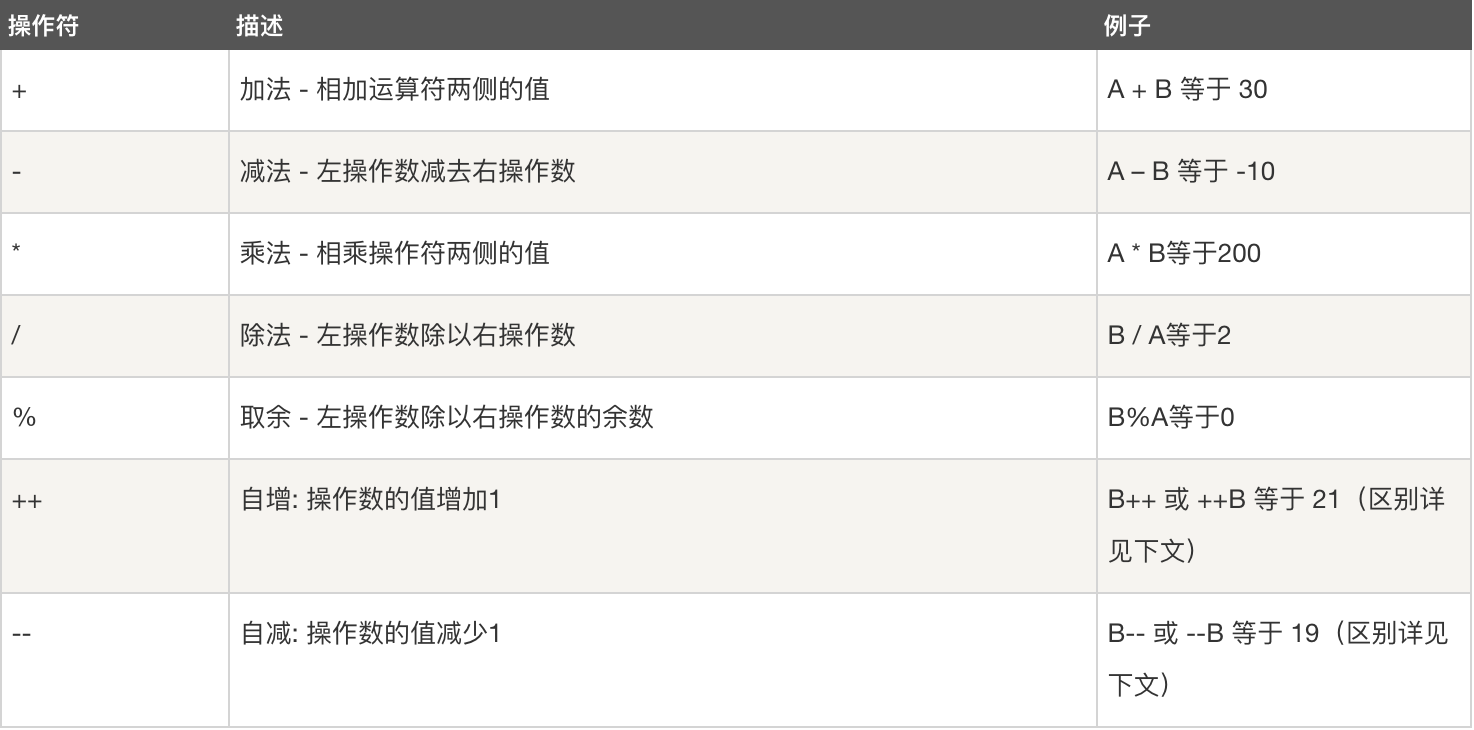### 实例

public class Test {

public static void main(String[] args) {
int a = 10;
int b = 20;
int c = 25;
int d = 25;
System.out.println("a + b = " + (a + b));
System.out.println("a - b = " + (a - b));
System.out.println("a * b = " + (a * b));
System.out.println("b / a = " + (b / a));
System.out.println("b % a = " + (b % a));
System.out.println("c % a = " + (c % a));
System.out.println("a++   = " + (a++));
System.out.println("a--   = " + (a--));
// 查看  d++ 与 ++d 的不同
System.out.println("d++   = " + (d++));
System.out.println("++d   = " + (++d));
}
}

a + b = 30
a - b = -10
a * b = 200
b / a = 2
b % a = 0
c % a = 5
a++   = 10
a--   = 11
d++   = 25
++d   = 27

### i++ 与 ++i 到底有什么不同？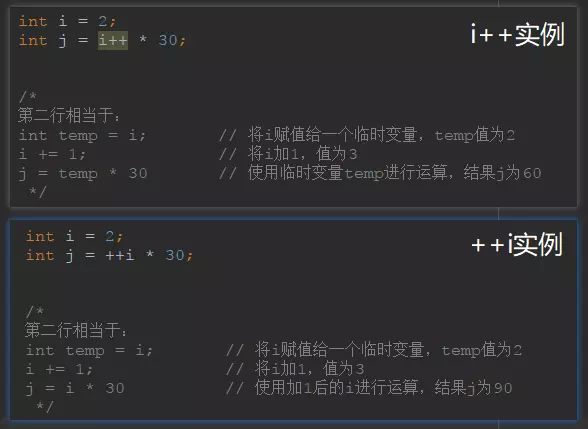## 关系运算符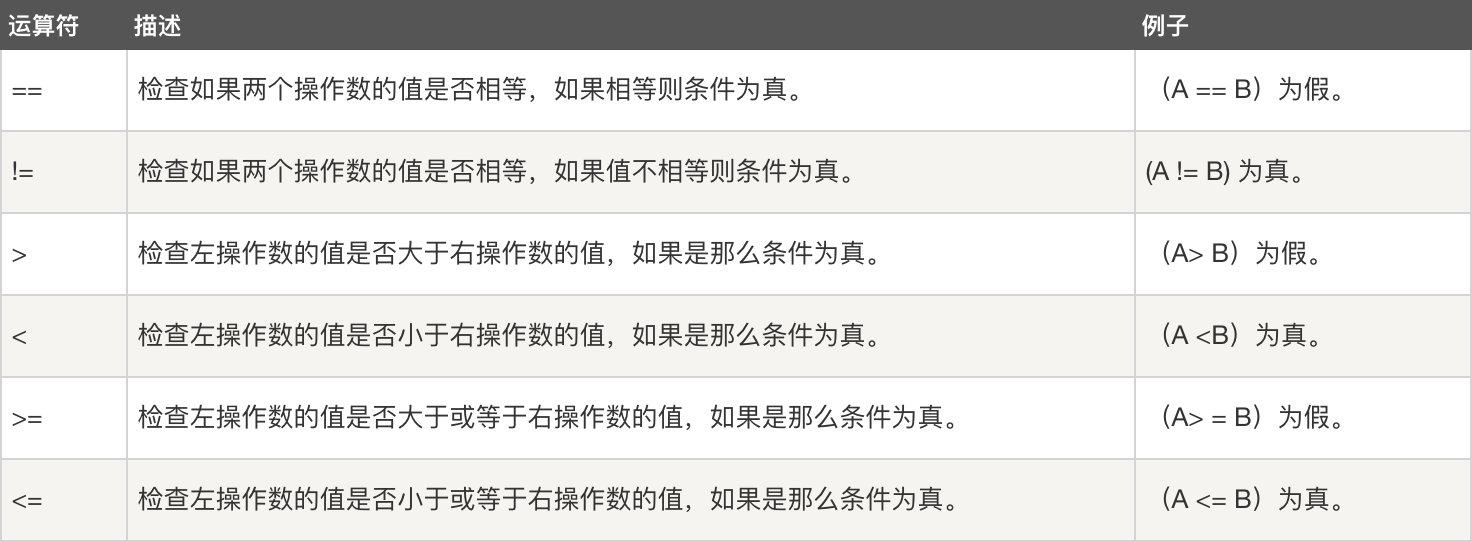### 实例

public class Test {

public static void main(String[] args) {
int a = 10;
int b = 20;
System.out.println("a == b = " + (a == b));
System.out.println("a != b = " + (a != b));
System.out.println("a > b = " + (a > b));
System.out.println("a < b = " + (a < b));
System.out.println("b >= a = " + (b >= a));
System.out.println("b <= a = " + (b <= a));
}
}

a == b = false
a != b = true
a > b = false
a < b = true
b >= a = true
b <= a = false

## 位运算符

Java定义了位运算符，应用于整数类型 (int)，长整型 *(long)，短整型 (short)，字符型 (char)，和字节型 *(byte) 等类型。

A = 0011 1100
B = 0000 1101
-----------------
A&B = 0000 1100
A | B = 0011 1101
A ^ B = 0011 0001
~A = 1100 0011### 实例

public class Test {

public static void main(String[] args) {
int a = 60; /* 60 = 0011 1100 */
int b = 13; /* 13 = 0000 1101 */
int c = 0;
c = a & b;       /* 12 = 0000 1100 */
System.out.println("a & b = " + c);

c = a | b;       /* 61 = 0011 1101 */
System.out.println("a | b = " + c);

c = a ^ b;       /* 49 = 0011 0001 */
System.out.println("a ^ b = " + c);

c = ~a;          /*-61 = 1100 0011 */
System.out.println("~a = " + c);

c = a << 2;     /* 240 = 1111 0000 */
System.out.println("a << 2 = " + c);

c = a >> 2;     /* 15 = 1111 */
System.out.println("a >> 2  = " + c);

c = a >>> 2;     /* 15 = 0000 1111 */
System.out.println("a >>> 2 = " + c);
}
} 

a & b = 12
a | b = 61
a ^ b = 49
~a = -61
a << 2 = 240
a >> 2  = 15
a >>> 2 = 15

## 逻辑运算符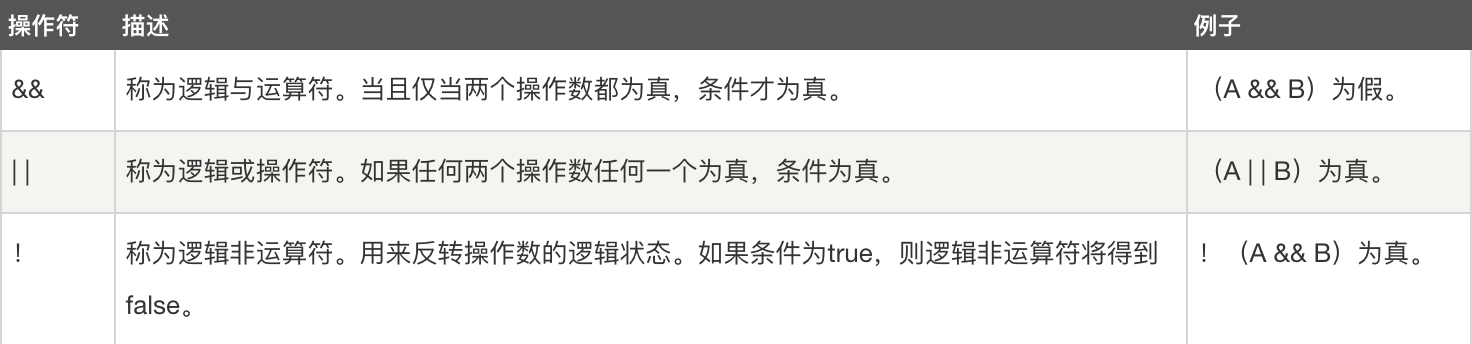### 实例

public class Test {

public static void main(String[] args) {
boolean a = true;
boolean b = false;
System.out.println("a && b = " + (a && b));
System.out.println("a || b = " + (a || b));
System.out.println("!(a && b) = " + !(a && b));
}
}

a && b = false
a || b = true
!(a && b) = true

### 短路逻辑运算符

public class LuoJi {

public static void main(String[] args) {
int a = 5;//定义一个变量；
boolean b = (a < 4) && (a++ < 10);
System.out.println("使用短路逻辑运算符的结果为" + b);
System.out.println("a的结果为" + a);
}
}

使用短路逻辑运算符的结果为false
a的结果为5

## 赋值运算符### 实例

public class Test {

public static void main(String[] args) {
int a = 10;
int b = 20;
int c = 0;
c = a + b;
System.out.println("c = a + b = " + c);
c += a;
System.out.println("c += a  = " + c);
c -= a;
System.out.println("c -= a = " + c);
c *= a;
System.out.println("c *= a = " + c);
a = 10;
c = 15;
c /= a;
System.out.println("c /= a = " + c);
a = 10;
c = 15;
c %= a;
System.out.println("c %= a  = " + c);
c <<= 2;
System.out.println("c <<= 2 = " + c);
c >>= 2;
System.out.println("c >>= 2 = " + c);
c >>= 2;
System.out.println("c >>= 2 = " + c);
c &= a;
System.out.println("c &= a  = " + c);
c ^= a;
System.out.println("c ^= a   = " + c);
c |= a;
System.out.println("c |= a   = " + c);
}
}

c = a + b = 30
c += a  = 40
c -= a = 30
c *= a = 300
c /= a = 1
c %= a  = 5
c <<= 2 = 20
c >>= 2 = 5
c >>= 2 = 1
c &= a  = 0
c ^= a   = 10
c |= a   = 10

## 其他运算符

### 条件运算符 (?:)

variable x = (expression) ? value if true : value if false

### 实例

public class Test {

public static void main(String[] args) {
int a, b;
a = 10;
// 如果 a 等于 1 成立，则设置 b 为 20，否则为 30
b = (a == 1) ? 20 : 30;
System.out.println("Value of b is : " + b);

// 如果 a 等于 10 成立，则设置 b 为 20，否则为 30
b = (a == 10) ? 20 : 30;
System.out.println("Value of b is : " + b);
}
}

Value of b is : 30
Value of b is : 20

### instanceof 运算符

instanceof 运算符使用格式如下：

( Object reference variable ) instanceof  (class/interface type)

String name = "James";
boolean result = name instanceof String; // 由于 name 是 String 类型，所以返回真

# 要点回顾

1. Java 是一种强类型语言，任何一种数据都属于 1 种基本类型或者对象类型 (类) 中的一种；
2. 8 种基本数据类型；
3. 为什么引入变量、如何定义使用变量以及变量名的命名规范；
4. Java 中的运算符以及使用实例；

# 练习

## 获取用户输入 Scanner

java.util.Scanner 是 Java5 的新特征，我们可以通过 Scanner 类来获取用户的输入。

Scanner scanner = new Scanner(System.in);

import java.util.Scanner;  // Import the Scanner class

class MyClass {
public static void main(String[] args) {
Scanner scanner = new Scanner(System.in);  // Create a Scanner object

}
}

### 输入类型## 练习 1：输入圆的半径计算周长和面积

import java.util.Scanner;

public class Tester {

public static void main(String[] args) {
System.out.println("请输入圆的半径：");

Scanner scanner = new Scanner(System.in);

// 计算周长和面积
double perimeter = 2 * 3.14 * radius;

System.out.println("该圆的面积为：" + area);
System.out.println("该圆的周长为：" + perimeter);
}
}

## 练习 2：输入年份判断是不是闰年

1. 能被 4 整除，并且不能被 100 整除；
2. 或者能被 400 整除；

import java.util.Scanner;

public class Tester {

public static void main(String[] args) {
System.out.println("请输入年份：");

Scanner scanner = new Scanner(System.in);
int year = scanner.nextInt();

boolean leapYear;

boolean divisbleBy4 = year % 4 == 0;
boolean notDivisbleBy100 = year % 100 != 0;
boolean divisibleBy400 = year % 400 == 0;
leapYear = (divisbleBy4 && notDivisbleBy100) || divisibleBy400;

System.out.println("该年份是否是闰年：" + leapYear);
}
}

# 自取资料

## 扩展阅读推荐

1. Java 基本数据类型 | 菜鸟教程 - https://www.runoob.com/java/java-basic-datatypes.html
2. 浮点类型精度深度讨论：
1. 为什么 0.1 + 0.2 = 0.3？ - https://draveness.me/whys-the-design-decimal-and-rational/
2. 为什么 0.1 + 0.2 = 0.300000004？ - https://draveness.me/whys-the-design-floating-point-arithmetic/#fn:2
3. IEEE754标准: 浮点数在内存中的存储方式 - https://www.jianshu.com/p/8ee02e9bb57d
4. 为什么Java中char类型不能完整表示一个字符？ - https://fookwood.com/java-string-charset-char

## 推荐书籍

#### Java 核心技术·卷 I(原书第 11 版)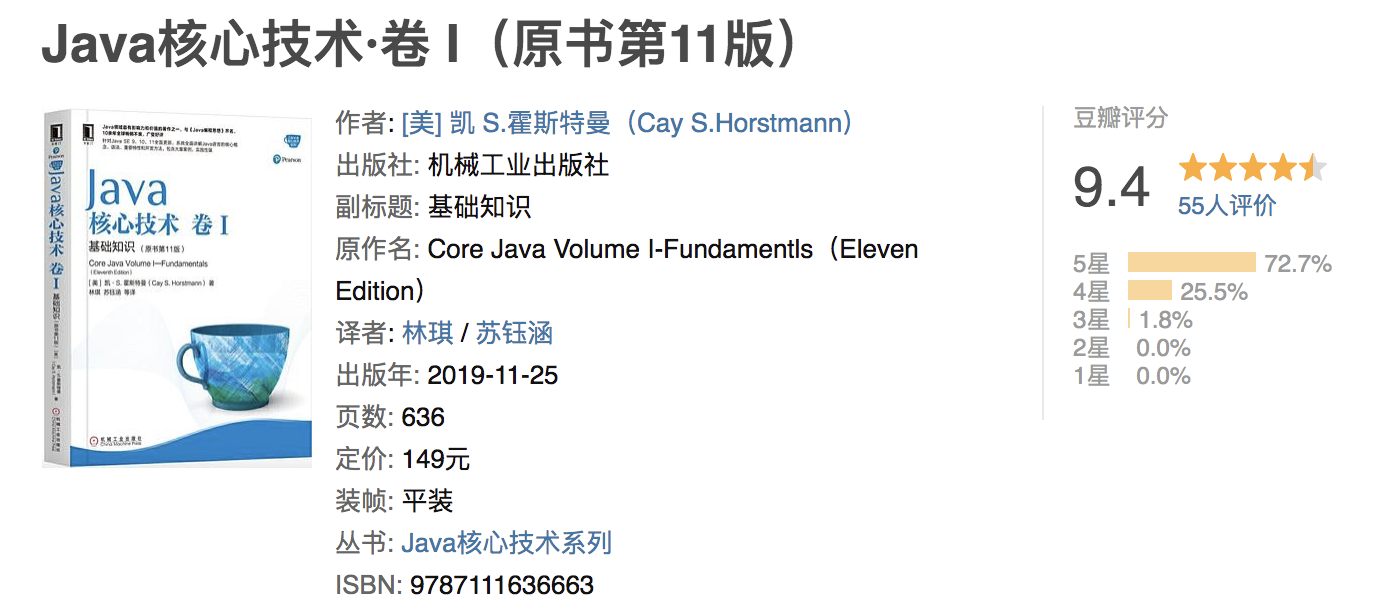#### 码出高效：Java开发手册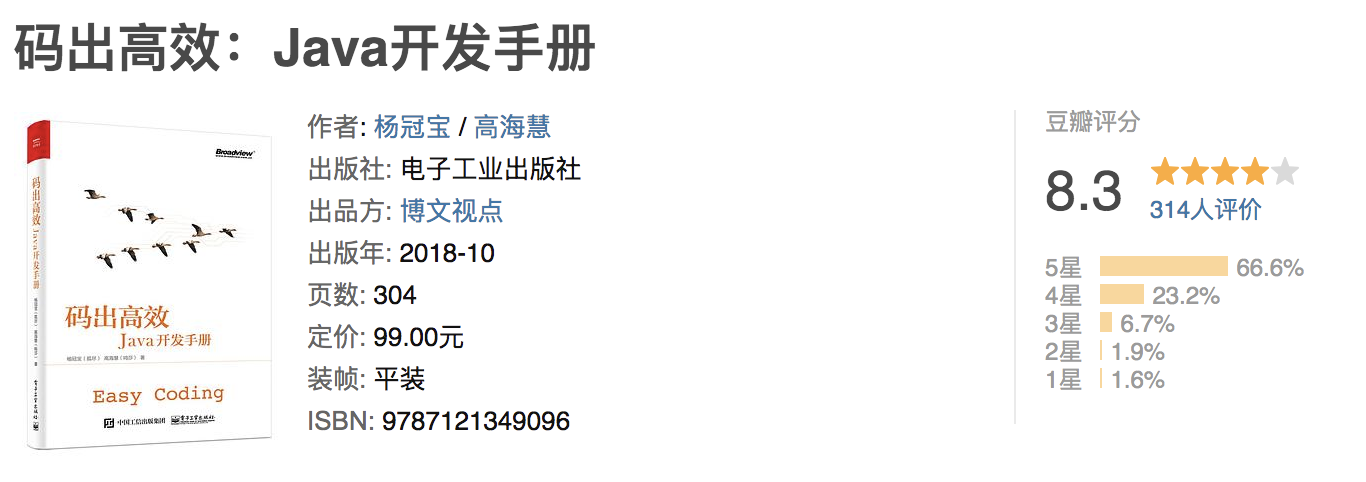# 参考资料

1. Introduction to Computer Science using Java - http://programmedlessons.org/Java9/index.html#part02
2. Java 运算符 | 菜鸟教程 - https://www.runoob.com/java/java-operators.html
3. 《Java 核心技术 卷I》(第11版)
• 本文已收录至我的 Github 程序员成长系列 【More Than Java】，学习，不止 Code，欢迎 star：https://github.com/wmyskxz/MoreThanJava
• 个人公众号 ：wmyskxz，个人独立域名博客：wmyskxz.com，坚持原创输出，下方扫码关注，2020，与您共同成长！